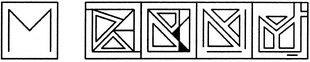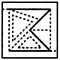# Non Verbal Reasoning - Embedded Images - Discussion

### Discussion :: Embedded Images - Section 2 (Q.No.5)

In each of the following questions, you are given a figure (X) followed by four alternative figures (1), (2), (3) and (4) such that figure (X) is embedded in one of them. Trace out the alternative figure which contains fig. (X) as its part.

5.

Find out the alternative figure which contains figure (X) as its part.(X)                (1)         (2)         (3)        (4)

 [A]. 1 [B]. 2 [C]. 3 [D]. 4

Explanation:Ashish Padhy said: (Feb 9, 2013) The embedded image in the choice given is rotated 90 degree clockwise. So the reason why option (a) is selected.

 Kat said: (Sep 27, 2020) Well said, Thanks @Ashish Padhy.